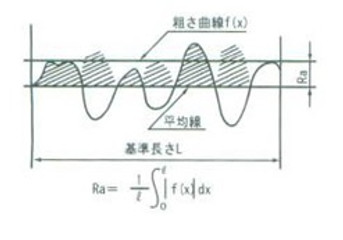## Technical data

Surface roughness measurement is an essential item not only for controlling the finished state and appearance quality, but also for managing product life and mechanical efficiency. In the evaluation of the plating film, the performance may be evaluated by measuring the surface roughness.

### Surface roughness

#### Maximum height Ry

Only the reference length is extracted from the roughness curve in the direction of the average line, the distance between the peak line and the valley bottom line of this extracted part is measured in the direction of the vertical magnification of the roughness curve, and this value is micrometer (μm). It is the one represented by.

Remarks: When calculating Ry, extract only the standard length from the part where there are no extraordinarily high peaks and low valleys that are considered to be scratches.#### Ten-point average roughness Rz

Only the reference length is extracted from the roughness curve in the direction of the average line, and the absolute value of the elevation (Yp) of the highest to fifth peaks measured in the direction of vertical magnification from the average line of this extracted part. The sum of the average value and the average value of the absolute values of the elevations (Yv) of the 5th valley bottom from the lowest valley bottom is calculated, and this value is expressed in micrometers (μm).#### Arithmetic Mean Roughness Ra

Extract only the reference length from the roughness curve in the direction of the average line, take the X axis in the direction of the average line of this extracted part, take the Y axis in the direction of the vertical magnification, and set the roughness curve to y = f (χ). When expressed in, the value obtained by the following formula is expressed in micrometer (μm).## Connection Co., Ltd.